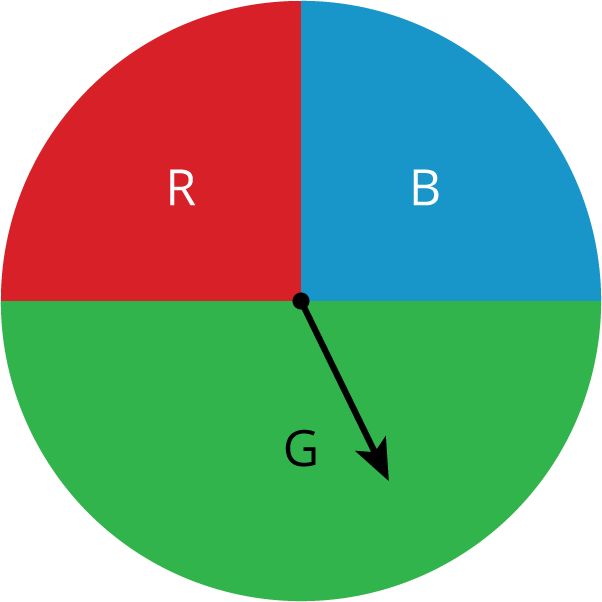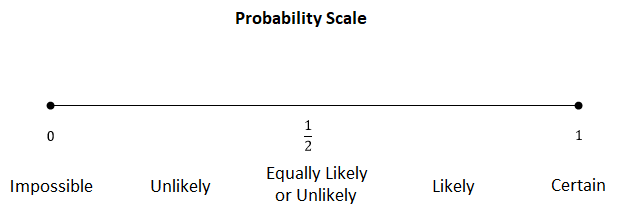# Probability

## Objective

Understand the probability of an event happening is a number between 0 and 1, ranging from impossible to certain.

## Common Core Standards

### Core Standards

?

• 7.SP.C.5 — Understand that the probability of a chance event is a number between 0 and 1 that expresses the likelihood of the event occurring. Larger numbers indicate greater likelihood. A probability near 0 indicates an unlikely event, a probability around 1/2 indicates an event that is neither unlikely nor likely, and a probability near 1 indicates a likely event.

## Criteria for Success

?

1. Understand that the probability of an event ranges from 0, impossible, to 1, certain, with various levels of likelihood in between.
2. Understand that an event that is equally likely or equally unlikely has a probability of about 0.5 or ${{1\over2}}$
3. Categorize and order the probabilities of events by their likelihood.
4. Use words like impossible, very unlikely, unlikely, equally likely/unlikely, likely, very likely, and certain to describe the probabilities of events.

## Tips for Teachers

?

This is students' first introduction to probability, however, they will probably have an informal vocabulary and understanding of what is “fair” or likely or unlikely. Make the connection between these understandings and the values, both decimal and fractional, between 0 and 1 that probabilities can take on. It may also be helpful to have an open discussion about what students know or think they know about probability before the lesson. This may help bring any misconceptions to the surface that can be addressed throughout the unit.

#### Fishtank Plus

• Problem Set
• Student Handout Editor
• Vocabulary Package

## Anchor Problems

?

### Problem 1

Ten events are described below. Decide which column in the table below each event best belongs to.

 Impossible Not Likely Equally Likely/Unlikely Likely Certain

Events:

1. You will win the grand prize in a raffle if you purchased 2 of the 100 tickets.2. You will wait less than 10 minutes before ordering at a fast-food restaurant.
3. You will get an even number when you roll a standard number cube.
4. A four-year-old child is over six feet tall.
5. No one in your class will be late to class next week.
6. The next baby born at a hospital will be a boy.
7. It will snow at our school on July 1.
8. The sun will set today before 11:00 p.m.
9. Spinning the spinner shown will result in green.
10. Spinning the spinner shown will result in yellow.

#### References

Open Up Resources Grade 7 Unit 8 Lesson 2 Teacher VersionActivity 2.2 "How Likely Is It?"

Modified by The Match Foundation, Inc.

### Problem 2

Order the following events from least likely to occur to most likely to occur. Explain your reasoning.

1. Twelve out of 20 students in your class are wearing glasses. The event that your teacher randomly calls on a student who is wearing glasses.
2. The weather report indicates a 90% chance of snow. The event that it snows.
3. The probability that Brand X of batteries is still working after 100 hours of use is 0.2. The event that a Brand X battery is still working after 100 hours of use.
4. You enter your name in a raffle for basketball tickets with 75 other people. Each person gets one entry per name. The event that you win the tickets.
5. A mouse running through a maze can turn left or right at the end of a path. The event that the mouse turns left.

### Problem 3

For each event below, design a spinner or a bag of cubes that would have the probabilities described.

1. The probability of selecting a red cube is 0.5.
2. The probability of spinning an even number is unlikely.
3. The probability of spinning a number greater than 3 is certain.
4. The probability of selecting a yellow cube is very likely.
5. The probability of spinning the color blue is 0.

## Problem Set

?The following resources include problems and activities aligned to the objective of the lesson that can be used to create your own problem set.

?

Decide where each of the following events would be located on the scale below. Place the letter for each event on the appropriate place on the probability scale.The numbers from 1 to 10 are written on small pieces of paper and placed in a bag. A piece of paper will be drawn from the bag.

1. A piece of paper with a 5 is drawn from the bag.
2. A piece of paper with an even number is drawn.
3. A piece of paper with a 12 is drawn.
4. A piece of paper with a number other than 1 is drawn.
5. A piece of paper with a number divisible by 5 is drawn.

#### References

EngageNY Mathematics Grade 7 Mathematics > Module 5 > Topic A > Lesson 1Exit Ticket

Grade 7 Mathematics > Module 5 > Topic A > Lesson 1 of the New York State Common Core Mathematics Curriculum from EngageNY and Great Minds. © 2015 Great Minds. Licensed by EngageNY of the New York State Education Department under the CC BY-NC-SA 3.0 US license. Accessed Dec. 2, 2016, 5:15 p.m..

?